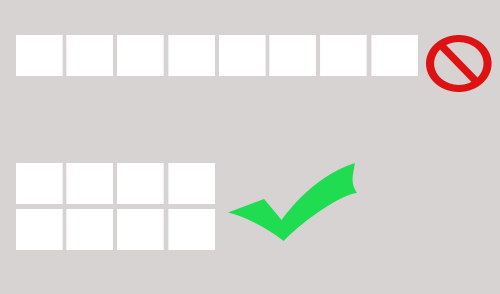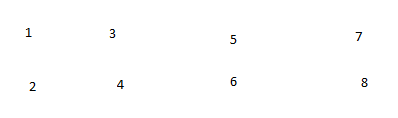# how to arrangement child objects using c#

i have a gameobject that have a group of plane meshes that created randomly as child every one of this planes have a “2f” offset in x axis between every plane

what i wanna do is i wanna make the parent object arrangement the child objects on rows and columns even if one of this child deleted during the game or added so if i have 8 planes mesh it should look like that 2 rows and 4 columns like that
but i don’t know how to do that ?i know how to get child objects and making offset but i do’t know how to get the format like that in the image
i wanna to arrangement the planes meshes that i can acces from this for loop

``````void Update(){
Transform[] children = new Transform[transform.childCount];

//Debug.Log(transform.childCount);
for (int i=0; i<transform.childCount; i++) {
children*=transform.GetChild(i);*
``````
• `````` 			}*
``````

}

Good day.

Then whats your question? How to place the meses ina 2 rows format?

The best solution i think is to create empty objects in the same positions where the planes will be. As this emptyobjects have a transfom, you can then just use its positions to place the planes.

``````plane1.transform.position = TheEmptyObject1. transform.position;
plane2.transform.position = TheEmptyObject2. transform.position;
....
``````

An depending on number of planes, decide where to put each oneBye!